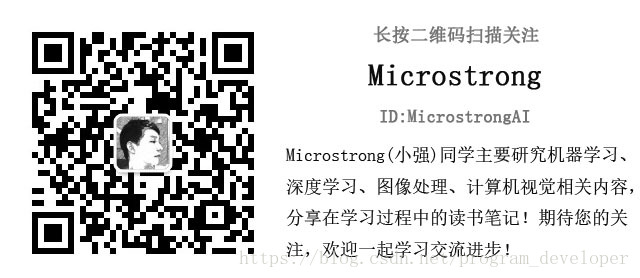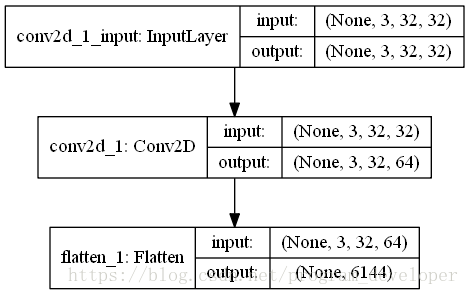# The function of flatten layer in deep learning

The official account of WeChatFlatten layer is implemented in Keras.layers.core . flatten() class.

effect:

Flatten layer is used to “flatten” the input, that is, to make the multi-dimensional input one-dimensional. It is often used in the transition from convolution layer to fully connected layer. Flatten does not affect the size of the batch.

example:

``````from keras.models import Sequential
from keras.layers.core import Flatten
from keras.layers.convolutional import Convolution2D
from keras.utils.vis_utils import plot_model

model = Sequential()
model.add(Convolution2D(64,3,3,border_mode="same",input_shape=(3,32,32)))
# now：model.output_shape==(None,64,32,32)

model.add(Flatten())
# now: model.output_shape==(None,65536)

plot_model(model, to_file='Flatten.png', show_shapes=True)``````

In order to better understand the function of flatten layer, I visualize this neural network, as shown in the figure below: